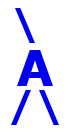## AIRES library reference: ghfl1jcint.

```c
c     Routine directly callable from C/C++ (it could be necessary to
c     append an underscore, "_", to its name depending on the C/C++
c     compiler used).
c
c
function ghfl1jcint(xmax, x0, lambda, jac)
c
c     Evaluating the complete integral of the Gaisser-Hillas function,
c     i.e.,
c
c            infty
c        I =  INT   Ngh(X) dX
c             X0
c
c     where Ngh(X) is a normalized Gaisser-Hillas function for the
c     profile of charged particles (T. K. Gaisser and A. M. Hillas,
c     in Proc. 15th ICRC (Plovdiv), vol. 8, p. 353 (1977)):
c
c                 /    X - X0   \ [(Xmax-X0)/lambda]
c        Ngh(X) = | ----------- |                    *
c                 \  Xmax - X0  /
c
c                       /  Xmax - X  \
c                 * exp | ---------- |
c                       \   lambda   /
c
c     for X > X0 (Ngh(X) = 0 for X < X0).
c
c     Written by: S. J. Sciutto, La Plata 2004.
c
c
c     Arguments:
c     =========
c
c     xmax, x0,
c     lambda.......... (input, double precision) The three parameters of
c                      the normalized Gaisser-Hillas function.
c                      Xmax, X0, and lambda are expressed in g/cm2.
c     jac............. (output, double precision, array(3)) The
c                      derivatives of the integral with respect to
c                      the three parameters of the normalized
c                      function.
c
c
c     Return value: (double precision) The value of the integral, or
c     ============  a large negative number in the case of invalid
c                   arguments.
c
c
c<--->
```

 These pages are maintained by Sergio J. Sciutto Back to AIRES library index Back to AIRES home